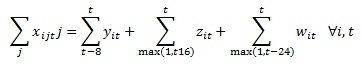## Help with coding

Hi,

I am trying to formulate this in SAS:How should i code this?

2 REPLIES 2

## Re: Help with coding

Your formula has a couple of issues, including overloading of t.  I assume you meant the following (in LaTeX notation):

\sum_j j x_{ijt} = \sum_{s=\max(1,t-8)}^t y_{is} + \sum_{s=\max(1,t-16)}^t z_{is} + \sum_{s=\max(1,t-24)}^t w_{is} \forall i,t

If so, you can write this constraint in PROC OPTMODEL as follows:

con Mycon {i in ISET, t in TSET}:
sum {j in JSET} j * x[i,j,t] = sum {s in max(1,t-8)..t} y[i,s] + sum {s in max(1,t-16)..t} z[i,s] + sum {s in max(1,t-24)..t} w[i,s];

## Re: Help with coding

Hi Rob,

Thanks for your help. I will give it a try.

Discussion stats
• 2 replies
• 834 views
• 0 likes
• 2 in conversation